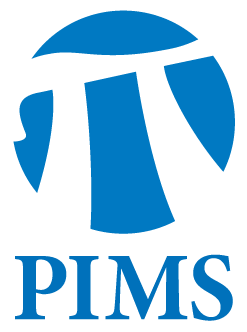## Number Theory Seminar: Kevin Doerksen

• Date: 12/01/2011
• Time: 15:00
Lecturer(s):
Kevin Doerksen
Location:

University of British Columbia

Topic:

On the prime divisors in zero orbits of families of commuting polynomials

Description:

Let (b_n) = (b_1, b_2, \dots) be a sequence of integers. A primitive prime divisor of the k-th term is a prime which divides b_k but does not divide any previous term in the sequence. A zero orbit of a polynomial f(x) is a sequence of integers (c_n) where the n-th term is the n-th iterate of f at 0. We consider primitive prime divisors of zero orbits
of polynomials and restrict to polynomials with zero linear term. In this talk, we show that if zero has an infinite orbit, then b_n will have primitive prime divisors for all n \geq 3.

We then consider a generalized notion of primitive prime divisors as it applies to families of commutative polynomials. We consider families of commutative polynomials which all have zero linear term and give an
effective bound on the number of terms in the forward orbit of 0 which do not have primitive prime divisors.

This talk is based on joint work with Jason Bell and builds on previous joint work with Anna Haensch.

Note for Attendees: Refreshments will be served between the two talks.

Schedule:

3:00-3:50pm in WMAX 216

Other Information:

Location: WMAX 216